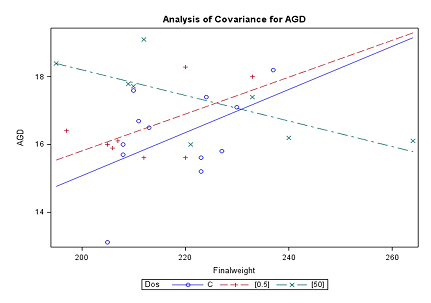10
Jan

–

10
Feb
Uppsala

# Statistics III: Regression analysis, 4hpThe Ph.D. course will be given in Uppsala 10 January - 10 February 2023.

#### Content

The course will cover the following topics:
• Simple linear regression.
• Multiple linear regression.
• Nonlinear models.
• Nonparametric regression and generalized additive models (GAM).
• Analysis of residuals.

#### Objectives

The objective of the course is to give an overview of linear, nonlinear and nonparametric regression. On completion of the course, the student will be able to:
• specify regression models including conditions and assumptions
• select an appropriate regression model for a given problem
• carry out a regression analysis in the statistical software R or SAS
• interpret and evaluate results correctly and draw reasonable conclusions
• clearly and concisely communicate results and conclusions

#### Prior knowledge

Statistics I: Basic Statistics or equivalent

## Contact